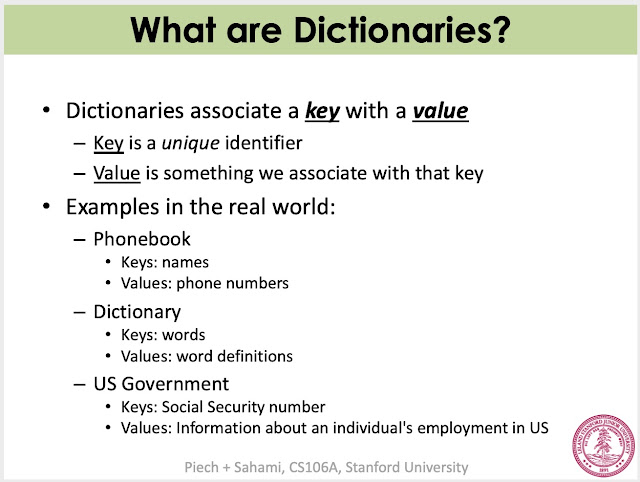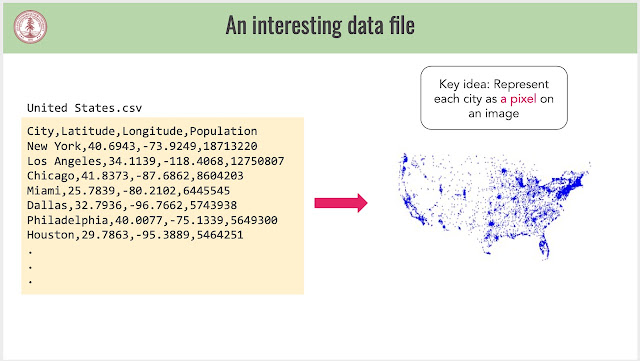# Stanford Python程式課 - 第五週

## 第五週學習目標Code in Place 第五週課程 Lesson 12: Dictionaries

## Lesson 12: Dictionaries 字典

### 學習重點

• None” - Python 用來代表 “no value”、
• 創建、使用、修改字典 Dictionary
• Dictionary 是 CodeinPlace 課程中最後學習的變數

### Dictionaries associate a key with a value

• Key: 獨一無二的指標
• Value: 與 key 關聯的內容Lecture 12-1: Intro

Lecture 12-2: Dictionaries

Lecture 12-4: Dictionaries are mutable

Lecture 12-5: Dictionary functions

Lecture 12-6: count_characters.py

Lecture 12-7: phone_book.py

## Lesson 13: Files 檔案

### 學習重點：

• 學習讀寫檔案
• 了解如何從檔案產生視覺化資料
• 學習 csv、json librariesLecture 13-1: Introduction

Lecture 13-2: What's a File?

Lecture 13-3: Reading and Writing Files

Lecture 13-4: Data Visualization

Lecture 13-5: Smarter Files

## Lesson 14: Data Science 資料科學

### 學習重點：

• 練習運用字典、清單、資料解決一些有趣的問題
• 終極 CS106A 問題 - 如何將一個字典反向？Google 搜尋快速是因為用了 Dictionary 架構

Lecture 14-1: Introduction

Lecture 14-2: Review

Lecture 14-3: Ultimate CS106A

Lecture 14-4: Ed forum (為何 Google 搜尋這麼快？)

### Dictionaries 程式範例

birthday.py

```"""
File: birthday.py
-----------------
Program to show an example of using dictionaries with functions.
"""

def have_birthday(dict, name):
"""
Print a birthday message and increment the age of the person
with the given name in the dictionary passed in.
"""
print("You're one year older, " + name + "!")
dict[name] += 1

def main():
ages = {'Chris': 33, 'Julie': 22, 'Mehran': 50}
print(ages)
have_birthday(ages, 'Chris')
print(ages)
have_birthday(ages, 'Mehran')
print(ages)

# Python boilerplate.
if __name__ == '__main__':
main()
```

count_characters.py

```"""
File: count_characters.py
-------------------------
This program counts the number of each character in the string TEXT.
It uses a dictionary to store the results, where each key is a
character and the corresponding value is the number of times that
character appeared in the string.
"""

TEXT = 'Happy day! I love the Code in Place community!'

def get_counts_dict(str):
"""
Returns a dictionary with where each key is a character and the corresponding
value is the number of times that character appeared in the string str passed in.
"""
counts = {}                             # create empty dictionary

for ch in str:
if ch not in counts:
counts[ch] = 1
else:
counts[ch] += 1

return counts

def print_counts(dict):
"""
This function prints out the key and its associated value for each
key/value pair in the dictionary passed in.
"""
print('Counts from dictionary')
for key in dict:
print(str(key) + ' = ' + str(dict[key]))

def main():
"""
Display the number of times each character appears in the constant TEXT.
"""
count_dict = get_counts_dict(TEXT)
print('count_dict = ', count_dict)
print_counts(count_dict)

# Python boilerplate.
if __name__ == '__main__':
main()
```

phonebook.py

```"""
File: phonebook.py
------------------
Program to show an example of using dictionaries to maintain
a phonebook.
"""

"""
Ask the user for names/numbers to story in a phonebook (dictionary).
Returns the phonebook.
"""
phonebook = {}  # Create empty phonebook

while True:
name = input("Name: ")
if name == "":
break
number = input("Number: ")
phonebook[name] = number

return phonebook

def print_phonebook(phonebook):
"""
Prints out all the names/numbers in the phonebook.
"""
for name in phonebook:
print(name, "->", phonebook[name])

def lookup_numbers(phonebook):
"""
Allow the user to lookup phone numbers in the phonebook
by looking up the number associated with a name.
"""
while True:
name = input("Enter name to lookup: ")
if name == "":
break
if name not in phonebook:
print(name + " is not in the phonebook")
else:
print(phonebook[name])

def main():
print_phonebook(phonebook)
lookup_numbers(phonebook)

# Python boilerplate.
if __name__ == '__main__':
main()
```

### Files 程式範例

plot_country.py:

```# don't worry about this import! It just allows us to grab all the files in the countries/ directory
import os

from simpleimage import SimpleImage

# Dimensions of the final visualization. Change these if the
# image is too large for your screen
VISUALIZATION_WIDTH = 1920
VISUALIZATION_HEIGHT = 1080

# Setting the 'boundaries' for the visualization. By default, the
# visualization holds the entire world. If you want to zoom in on a
# specific country, you can find the corresponding latitudes and longitudes
# here: https://gist.github.com/graydon/11198540
MIN_LONGITUDE = -180
MAX_LONGITUDE = 180
MIN_LATITUDE = -90
MAX_LATITUDE = 90

# The folder in which all the country data can be found
COUNTRY_DIRECTORY = "countries/"

def plot_country(visualization, filename):
"""
Responsible for reading in geographic data from a file
about the cities in a particular country and plotting them
in the visualization.

Parameters:
- `visualization` is the SimpleImage that will eventually be
shown to the user
- `filename` is the file we want to read through
"""
# TODO fill me in!
with open(filename) as f:
next(f)
for line in f:
line = line.strip()
parts = line.split(",")
latitude = float(parts)
longtitude = float(parts)

plot_one_city(visualization, latitude, longtitude)

"""
DO NOT MODIFY THE CODE BELOW

(but you're welcome to read it 😀 )
"""

def main():
# create a blank image on which we'll plot cities
visualization = SimpleImage.blank(
VISUALIZATION_WIDTH, VISUALIZATION_HEIGHT
)

# get which countries should be plotted from the user
countries = get_countries()

# iterate through each of the countries and plot it
for country in countries:
country_filename = COUNTRY_DIRECTORY + country + ".csv"
plot_country(visualization, country_filename)

# once we're done with all the countries, show the image
visualization.show()

def get_countries():
"""
Gets the list of countries from the user, but doesn't check that
the user types in valid country names.

Returns a list of country names
"""
countries = []
while True:
country = input("Enter a country, or 'all'. Press enter to finish: ")
if country == "":
break
if country == "all":
# `COUNTRY_DIRECTORY` and returns a list of all the filenames
return [s.split(".") for s in os.listdir(COUNTRY_DIRECTORY)]

# if the user didn't press enter immediately or type all,
# store the country name

# Add this line so country name of lower case will also work
country = country.title()

countries.append(country.strip())

return countries

def plot_one_city(visualization, latitude, longitude):
"""
Given the visualization image as well as a single city's latitude and longitude,
plot the city on the image

Parameters:
- `visualization` is the SimpleImage that will eventually be
shown to the user
- `latitude` is the latitude of the city (a float)
- `longitude` is the longitude of the city (a float)
"""

# convert the Earth coordinates to pixel coordinates
x = longitude_to_x(longitude)
y = latitude_to_y(latitude)

# if the pixel is in bounds of the window we specified through constants,
# plot it
if 0 < x < visualization.width and 0 < y < visualization.height:
plot_pixel(visualization, x , y)

def plot_pixel(visualization, x, y):
"""
Set a pixel at a particular coordinate to be blue. Pixels start off as
white, so all three color components have a value of 255. Setting the red
and green components to 0 makes the pixel appear blue.

Note that we don't return anything in this function because the Pixel is
'mutated' in place

Parameters:
- `visualization` is the SimpleImage that will eventually be
shown to the user
- `x` is the x coordinate of the pixel that we are turning blue
- `y` is the y coordinate of the pixel that we are turning blue
"""
pixel = visualization.get_pixel(x, y)
pixel.red = 0
pixel.green = 0

def longitude_to_x(longitude):
"""
Scales a longitude coordinate to a coordinate in the visualization email
"""
return VISUALIZATION_WIDTH * (longitude - MIN_LONGITUDE) / (MAX_LONGITUDE - MIN_LONGITUDE)

def latitude_to_y(latitude):
"""
Scales a latitude coordinate to a coordinate in the visualization email
"""
return VISUALIZATION_HEIGHT * (1.0 - (latitude - MIN_LATITUDE) / (MAX_LATITUDE - MIN_LATITUDE))

if __name__ ==  "__main__":
main()```

write_example.py:

```"""
Example of using library to write CSV
"""
import csv

def write_data():
with open("data.csv", "w") as f:
writer = csv.writer(f)
writer.writerow(["x", "y"])
writer.writerows([
[1,2],
[2,4],
[4,6]
])

def main():
write_data()

if __name__ == "__main__":
main()
```

dictwriter_example.py:

```"""
Example of using dictionary to write CSV
"""
import csv

def write_data():
with open("data.csv", "w") as f:
columns = ['x', 'y']
writer = csv.DictWriter(f, fieldnames=columns)
writer.writerow({'x': 1, 'y': 2})
writer.writerow({'x': 2, 'y': 4})
writer.writerow({'x': 4, 'y': 6})

def main():
write_data()

if __name__ == "__main__":
main()
```

### Data 程式範例

ed_small.json (資料檔) :

```[
{
"created_at": "2021-05-21T01:20:39.296044+10:00",
"user": {
"name": "Anonymous",
"role": ""
}
},
{
"created_at": "2021-05-21T01:21:25.225994+10:00",
"user": {
"name": "Anonymous",
}
},
{
"created_at": "2021-05-21T01:18:55.160661+10:00",
"user": {
"name": "Anonymous",
"role": ""
}
},
{
"created_at": "2021-05-21T01:29:58.2526+10:00",
"user": {
"name": "Anonymous",
"role": ""
}
},
{
"created_at": "2021-05-21T01:02:48.854092+10:00",
"user": {
"name": "Anonymous",
"role": ""
}
},
{
"created_at": "2021-05-20T23:57:12.340442+10:00",
"user": {
"name": "Anonymous",
"role": ""
}
},
{
"created_at": "2021-05-20T23:40:06.718832+10:00",
"user": {
"name": "Anonymous",
"role": ""
}
},
{
"created_at": "2021-05-21T00:21:04.357038+10:00",
"user": {
"name": "Anonymous",
"role": ""
}
},
{
"created_at": "2021-05-21T00:11:00.373241+10:00",
"user": {
"name": "Anonymous",
}
},
{
"created_at": "2021-05-20T23:18:03.604335+10:00",
"user": {
"name": "Anonymous",
"role": ""
}
}
]
```

post_times.py:

```# for loading files with nested data
import json
# for turning strings into dates
from dateutil import parser
from pytz import timezone
# for making pretty graphs
import seaborn as sns
import matplotlib.pyplot as plt

def main():

hour_counts = {}
for hour in range(24):
hour_counts[hour] = 0

for post in ed_data:
timestamp = post['created_at']
hour = get_hour(timestamp)
hour_counts[hour] += 1

print('day, n_posts')
for hour in range(24):
n_posts = hour_counts[hour]
print(hour, n_posts)
make_bar_plot(hour_counts)

def get_hour(time_string):
"""
Given a time string, returns the day of the week (in pacific time).
>>> get_hour('2021-05-21T01:20:39.296044+10:00')
'Thu'
"""
date_time = parser.parse(time_string)
# change to my timezone
date_time = date_time.astimezone(timezone('US/Pacific'))
# get the hour out of the time object
return date_time.hour

def make_bar_plot(count_map):
"""
Turns a dictionary (where values are numbers) into a bar plot.
Labels gives the order of the bars! Uses a package called seaborn
for making graphs.
"""
# turn the counts into a list
counts = []
# loop over the labels, in order
for label in count_map:
counts.append(count_map[label])
# format the data in the way that seaborn wants
data = {
'x':list(count_map.keys()),
'y':counts
}
sns.barplot(x = 'x',y = 'y', data= data)
plt.savefig("plot.png")

if __name__ == '__main__':
main()
```

student_to_staff.py:

```# for loading files with nested data
import json

def main():

n_teacher_posts = 0
# loop over each post in the list
for post in ed_data:
# get the role of the author of the post
role_str = post['user']['role']
# if the post came from a teacher
if role_str == 'tutor' or role_str == 'admin':
# increase the count
n_teacher_posts += 1

# show the fraction of posts from teachers
n_posts = len(ed_data)
print(n_teacher_posts / n_posts)

if __name__ == '__main__':
main()
```